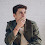Only Engineers Can Solve This Puzzle. Try if you can.

1 + 4  = 5
2 + 5 = 12
3 + 6  = 21
5 + 8 = ??

SHARE IF YOU GOT IT

44
(5 x 8) + 4

1.How to get 44, 1+4=5 4×1=4+1=5,2+5=12 2×5=10+2=12,3+6=21 3×6=18+3=21,missing 4+7=32 7×4=28+4=32, 8+5=45 8×5=40+5=45

1.You guys are overthinking this series! Where are you assuming multiplication is a valid operator, whereby you're constructing your answer by such use?

2.45

3.45

4.1.2.34 is correct! This is an addition problem - there are no references to using multiplication as the respective operator. To get 45 as an answer, you are assuming you can CHANGE the operator from "+" to "x" which is a different series operation altogether.

5.45 is correct

6.7.8.1+4=5;2+5=7+5=12;3+6=9+12=21;5+8=13+21=34

1.2.9.10.11.12.13.